# Square root of 2

• Last Updated : 08 Oct, 2020

Square root of a number is a positive algebraic expression represented by the symbol ‘‘ and is written as √x or x½. Square root of 2 is an irrational number represented as √2 or 2½. It is when multiplied by itself, will result in the number 2. The value of this expression i.e. √2 is 1.414… Its value can’t be determined exactly because it can not be represented as a fraction i.e. in the form of a/b where a and b are whole numbers and has an infinite number of decimals.

The value of Square root of 2 is widely used in mathematics as 1.414 because it contains an infinite number of decimals. Hence, to make mathematical computations easy, we use only 3 digits after decimal places. Sometimes 99/70 is also used as a value for Square root of 2.

Hey! Looking for some great resources suitable for young ones? You've come to the right place. Check out our self-paced courses designed for students of grades I-XII

Start with topics like Python, HTML, ML, and learn to make some games and apps all with the help of our expertly designed content! So students worry no more, because GeeksforGeeks School is now here!

#### Computing Square Roots

The square root of a number is the value which when multiplied by itself, results in the number taken as input. To compute the square root, we first need to check if the number is a perfect square. Perfect Squares are the numbers, the roots of which are whole numbers. For example, 4, 9, 25, 36, 49, etc. It is easier to compute the square root of a perfect square number as compared to a non-perfect square number. To compute the root of a non-perfect number, we need to apply the formula of the long division method.

#### How to perform the Long Division Method?

To compute the square root of a number using the long division method, go through the steps given below:

Step 1: First we need to divide the number into groups of two starting from right to left.

For example: To compute the value of √132496, we divide the digits into groups like 13, 24, and 96.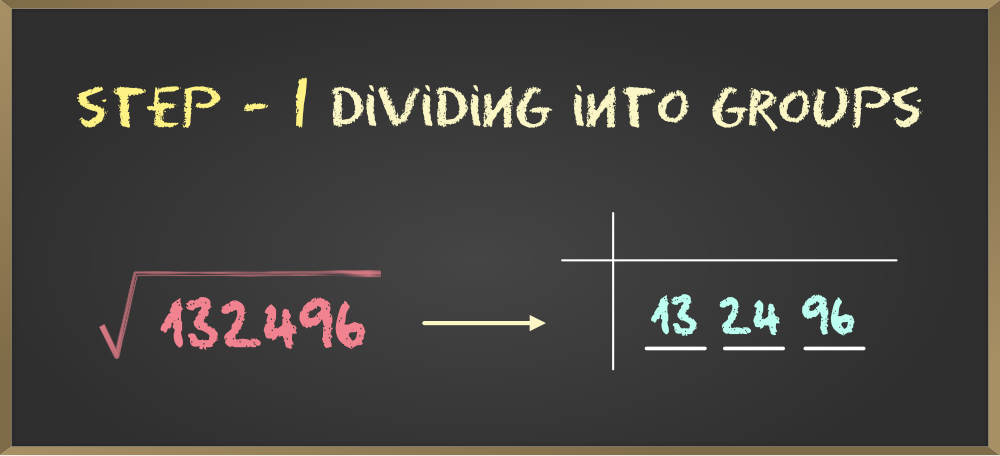Step 2: Now we need to find the highest number that when multiplied by itself results in the number less than or equal to the first pair of digits.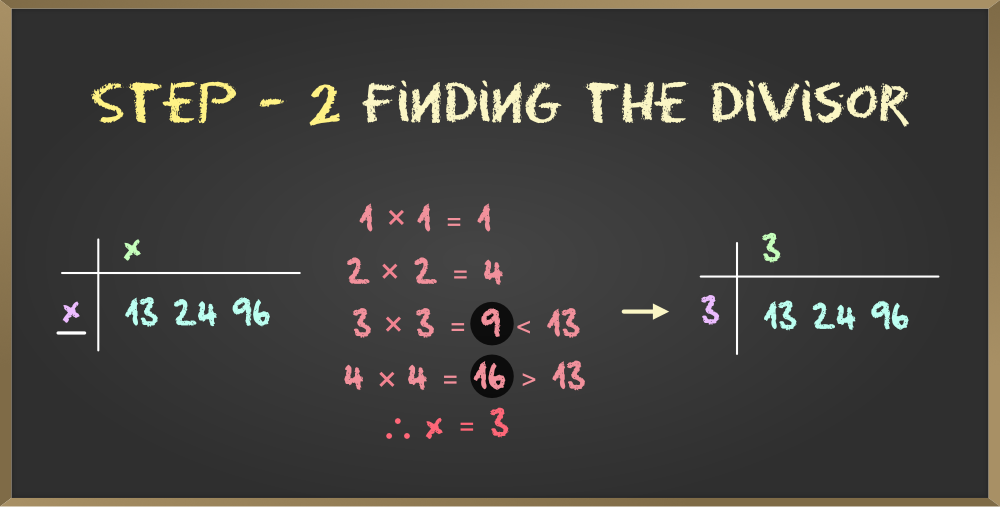Here, we need a number that when multiplied by itself results in product ≤ 13. So, the highest number that can be selected is 3.

Step 3: Now compute the remainder and then write the next pair of digits next to the remainder. This will become our dividend for the next step.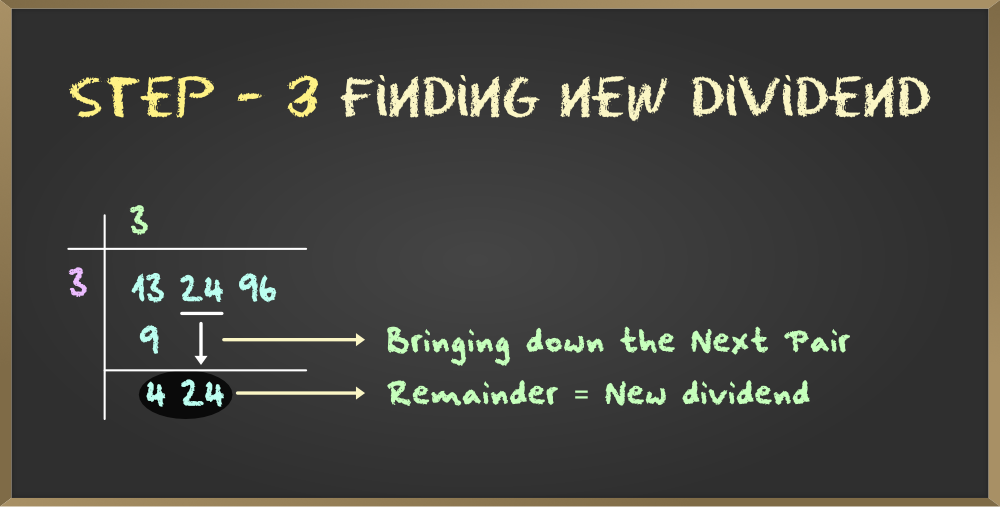Step 4: To create the divisor, first we will multiply the quotient with 2 and write the product as the digit for tens place of the divisor. For the unit’s place, we will again perform Step 2.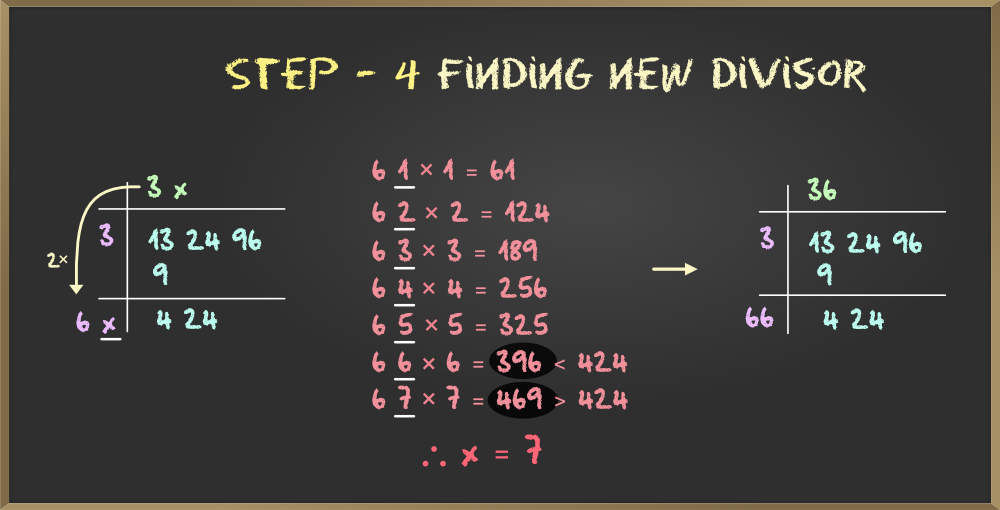Step 5: Now perform Step 3 and Step 4 again and then again repeat Step 2 to create the divisor. Continue the same until the remainder becomes zero.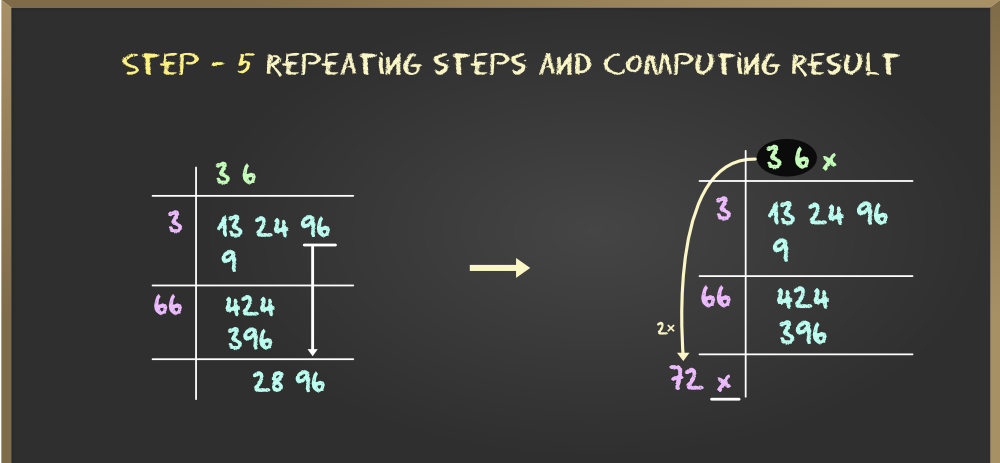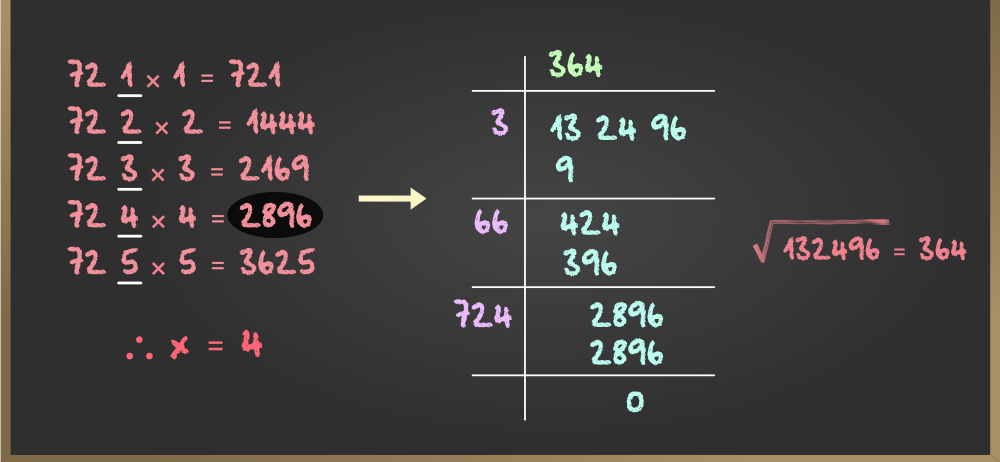The quotient formed will be the square root of the number.

#### How to Find Square Root of 2?

It is always easier to compute the square root of perfect squares but to compute square root of a non-perfect square, we need to perform the long division method. To compute the square root of 2, we need to follow the steps given below:

Step 1: Write 2 as 2.000000 to make it easier to divide

Step 2: Now look for the perfect square less than 2 i.e. 1 and divide the number with it.

Step 3: Now the quotient and the remainder are 1. We will place a decimal in the quotient and bring down the pair of zeroes for further division.

Step 4: Now add the quotient with the existing divisor, this will become the digit at the tens place for out next divisor.

Step 5: For the units place, we need to find a value that can be placed at the unit place of both quotient and the divisor such that the new divisor when multiplied with the unit digit of the quotient results in the highest number less than the remainder.

Now. bring down the next pair of zeros and repeat the steps 4th and 5th. This can be done for infinite steps as the exact value of the square root of 2 goes up to infinite decimal places. We can compute the result up to 4 decimal places as that can be used for approx. value of the square root.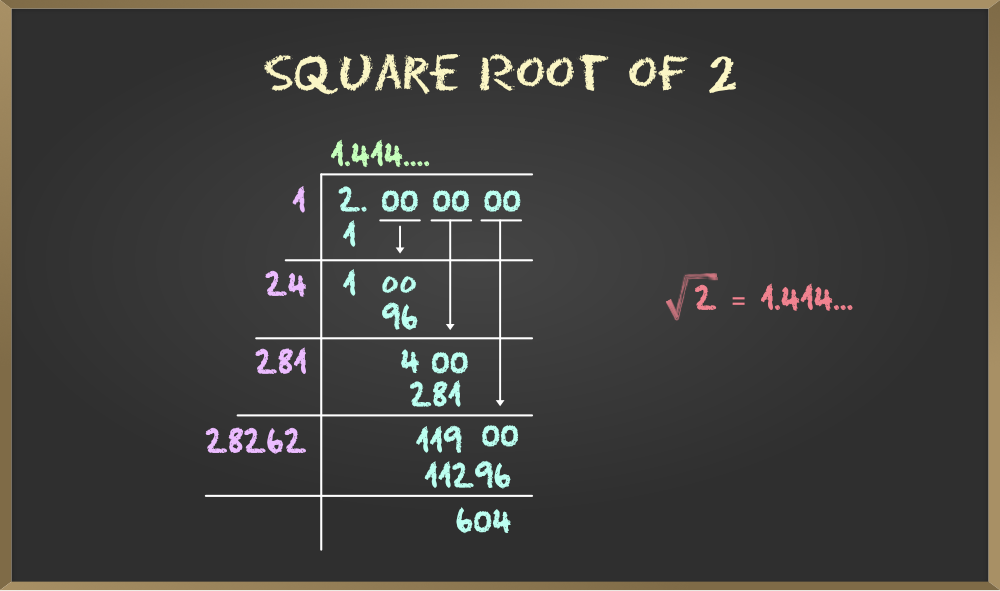My Personal Notes arrow_drop_up